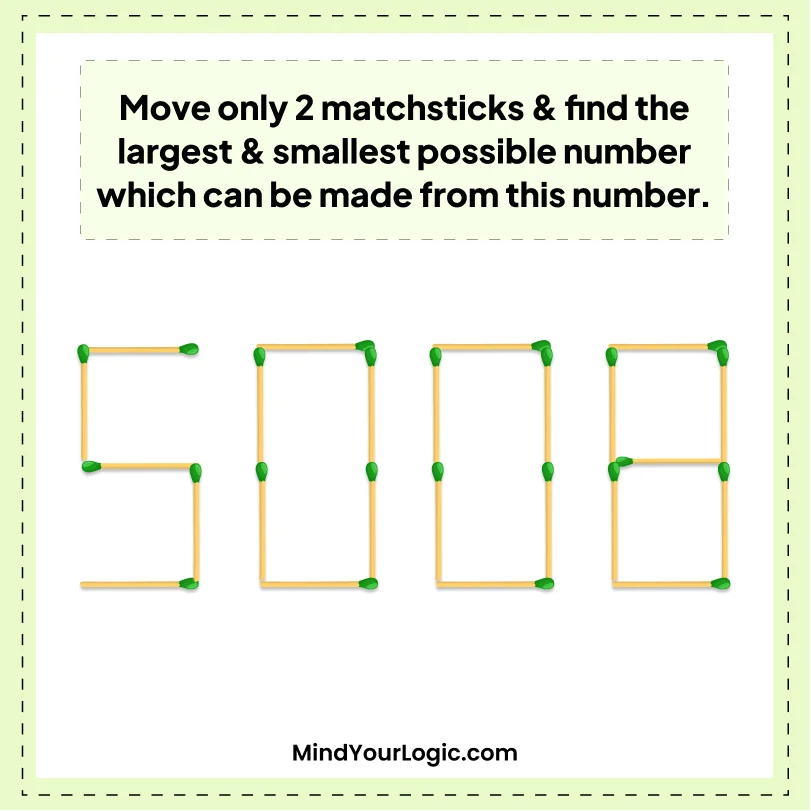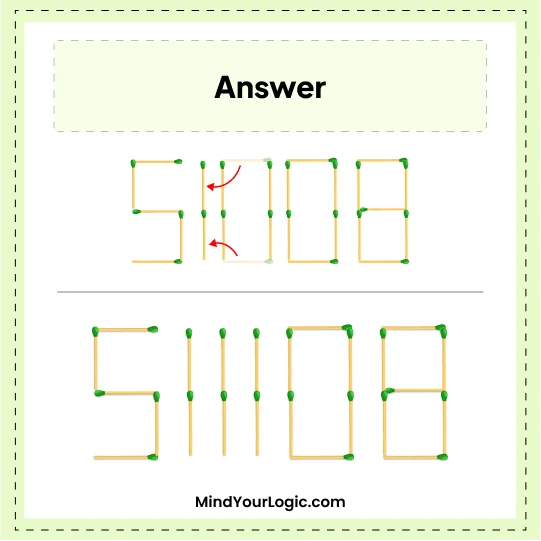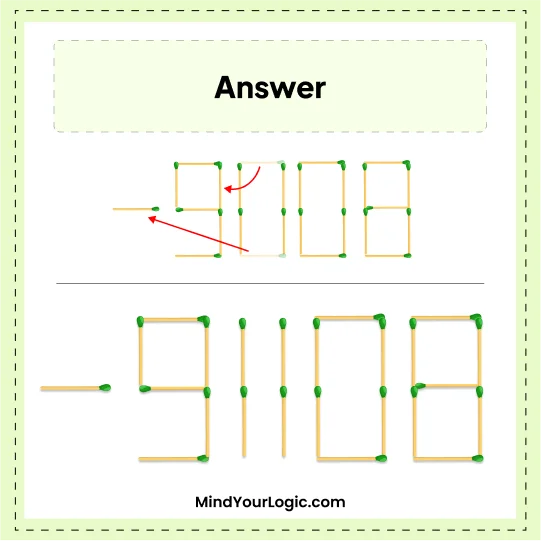# Largest and Smallest Number from 508 - Matchsticks Puzzle

###### 12.Matchstick Puzzles
`Move only 2 matchsticks and find the largest & smallest possible number which can be made from this number.`•

Explanation :

``` The Largest Number would be 511108.
Move two horizontal matchsticks from frist zeros, as a result, it becomes 11.
Then, create 1 between 11 using the matchsticks which were removed to make 111 as shown in the below image.

The Smallest Number would be -91108.
Move one from 111 matchsticks as a result it becomes 11. Then, using the two matchsticks which were removed, use one of them to make 9 out of 5 and use the second one for the ?- ?sign in front of 9.
```